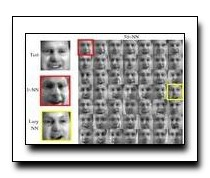Local Distance Functions: A Taxonomy, New Algorithms, and an Evaluation
Deva Ramanan, Simon BakerWe present a taxonomy for local distance functions where most existing algorithms can be regarded as approximations of the geodesic distance defined by a metric tensor. We categorize existing algorithms by how, where and when they estimate the metric tensor. We also extend the taxonomy along each axis. How: We introduce hybrid algorithms that use a combination of dimensionality reduction and metric learning to ameliorate over-fitting. Where: We present an exact polynomial time algorithm to integrate the metric tensor along the lines between the test and training points under the assumption that the metric tensor is piecewise constant. When: We propose an interpolation algorithm where the metric tensor is sampled at a number of references points during the offline phase, which are then interpolated during online classification. We also present a comprehensive evaluation of all the algorithms on tasks in face recognition, object recognition, and digit recognition.

### Text Reference

Deva Ramanan and Simon Baker. Local distance functions: a taxonomy, new algorithms, and an evaluation. In International Conference on Computer Vision (ICCV). 2009.

### BibTeX Reference

@inproceedings{RamananB_ICCV_2009,
author = "Ramanan, Deva and Baker, Simon",
booktitle = "International Conference on Computer Vision (ICCV)",
title = "Local Distance Functions: A Taxonomy, New Algorithms, and an Evaluation",
year = "2009",
tag = "object_recognition"
}